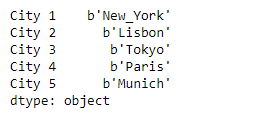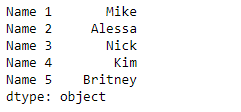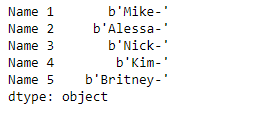# Python | Pandas Series.str.encode()

`Series.str` can be used to access the values of the series as strings and apply several methods to it. Pandas` Series.str.encode()` function is used to encode character string in the Series/Index using indicated encoding. Equivalent to `str.encode()`.

Syntax: Series.str.encode(encoding, errors=’strict’)

Parameter :
encoding : str
errors : str, optional

Returns : encoded : Series/Index of objects

Example #1: Use `Series.str.encode()` function to encode the character strings present in the underlying data of the given series object. Use ‘raw_unicode_escape’ for encoding.

 `# importing pandas as pd ` `import` `pandas as pd ` ` `  `# Creating the Series ` `sr ``=` `pd.Series([``'New_York'``, ``'Lisbon'``, ``'Tokyo'``, ``'Paris'``, ``'Munich'``]) ` ` `  `# Creating the index ` `idx ``=` `[``'City 1'``, ``'City 2'``, ``'City 3'``, ``'City 4'``, ``'City 5'``] ` ` `  `# set the index ` `sr.index ``=` `idx ` ` `  `# Print the series ` `print``(sr) `

Output :Now we will use `Series.str.encode()` function to encode the character strings present in the underlying data of the given series object.

 `# use 'raw_unicode_escape' encoding ` `result ``=` `sr.``str``.encode(encoding ``=` `'raw_unicode_escape'``) ` ` `  `# print the result ` `print``(result) `

Output :As we can see in the output, the `Series.str.encode()` function has successfully encoded the strings in the given series object.

Example #2 : Use `Series.str.encode()` function to encode the character strings present in the underlying data of the given series object. Use ‘punycode’ for encoding.

 `# importing pandas as pd ` `import` `pandas as pd ` ` `  `# Creating the Series ` `sr ``=` `pd.Series([``'Mike'``, ``'Alessa'``, ``'Nick'``, ``'Kim'``, ``'Britney'``]) ` ` `  `# Creating the index ` `idx ``=` `[``'Name 1'``, ``'Name 2'``, ``'Name 3'``, ``'Name 4'``, ``'Name 5'``] ` ` `  `# set the index ` `sr.index ``=` `idx ` ` `  `# Print the series ` `print``(sr) `

Output :Now we will use `Series.str.encode()` function to encode the character strings present in the underlying data of the given series object.

 `# use 'punycode' encoding ` `result ``=` `sr.``str``.encode(encoding ``=` `'punycode'``) ` ` `  `# print the result ` `print``(result) `

Output :As we can see in the output, the `Series.str.encode()` function has successfully encoded the strings in the given series object.

Whether you're preparing for your first job interview or aiming to upskill in this ever-evolving tech landscape, GeeksforGeeks Courses are your key to success. We provide top-quality content at affordable prices, all geared towards accelerating your growth in a time-bound manner. Join the millions we've already empowered, and we're here to do the same for you. Don't miss out - check it out now!

Previous
Next